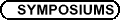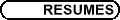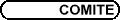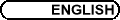Contributed Papers Session / Communications libres
Org: William G. Brown (McGill)

RYUICHI ASHINO, Mathematical Sciences, Osaka Kyoiku University, Kashiwara, Osaka 582-8582, Japan
A pre-processing design for the discrete multiwavelet transform of irregularly sampled data

Unshifted and shifted multiscaling functions are used as mathematical models for curve fitting of irregularly sampled data. A pre-processing design for the discrete multiwavelet transform based on this curve fitting method is proposed. This pre-processing procedure combined with multiwavelet neural networks for data-adaptive curve fitting is shown to perform well in the case of high resolution. In the case of low resolution it is more accurate than numerical integration and cheaper than matrix inversion.

This is joint work with Akira Morimoto and Rémi Vaillancourt.

CHANTAL BUTEAU, Brock University, St. Catharines, Ontario L2S 3A1
Topological Spaces of Music Motives: An Application of T0-Spaces

We present a topological model of motivic analysis of music that mainly consists of identifying in a music composition the short sequence of notes melodically generating, by its repetitions and variations, the whole score. Our approach particularly solves the problem of motif similarity for different cardinalities. Using the music concepts of melodic contour-map on the set of all possible motives, of gestalt-orbit of a motif for a given group action, and of motif similarity-pseudo-metric for motives with same cardinality, we introduce a topological structure on the set of all motives. The crucial step in our approach is the definition of motif neighborhoods that links motives with different cardinalities. In the resulting T0-space, the identification of the wanted germinal (generator) motif corresponds to the determination of the motif with most `dense' neighborhood given a similarity threshold. We illustrate our approach with Bach's "Art of Fugue" for which we investigated the still debated problem on the length of its main theme. Others' approaches based on the so-called (music) Set Theory can be consistently redefined and extended in our generic topological structure.

This model is part of the local theory of the so-called Mathematical Music Theory developed by G. Mazzola in his book The Topos of Music-Geometric Logic of Concepts, Theory, and Performance, and which is based on local charts and global discrete varieties of tones in specific parameter spaces.

ADELA N. COMANICI, University of Houston, Department of Mathematics
Transition from Rotating Waves to Modulated Rotating Waves on a Sphere

Spiral waves are spatio-temporal patterns that have been observed in numerous physical situations, ranging from Belousov-Zhabotinsky chemical reactions to cardiac tissue. The global geometry of the heart is closer to a sphere than to a plane. Therefore, it would be important to study spiral wave dynamics in a context where the symmetries are that of a sphere instead of a plane. Also, rigidly rotating spiral waves are rotating waves. In this talk we consider an SO(3)-equivariant reaction-diffusion system on a sphere:

 ¶u ¶t (t,x) = DDu (t,x) + F æè u(t,x), l öø on r S2,
(1)
u = (u1,u2,...,uN) with N ³ 1, D is the diffusion matrix and F = (F1,F2,...,FN) is a sufficiently smooth function.

For l = 0 we consider a relative equilibrium SO(3) u0 with trivial isotropy Su0 = I3 consisting of rotating waves. By Hopf bifurcation from rotating waves we generically get modulated rotating waves. We show that there exists a decomposition of these modulated rotating waves in two parts: a primary frequency vector part, eX(l)t and a [(2p)/(|wl|)]-periodic part, B(t,l). We present a way of contructing X(l) and B(t,l) using the BCH formula in SO(3) and the solution Z(t,l) to the initial value problem on some interval [0,[(2p)/(n|wl|)]], with integer n > 0 independent of l:

 ® Z¢
= é
ë
I3 +  1

2
Z+ æ
è
1

|Z|2
-
 cos |Z| 2

 2sin |Z| 2 |Z|
ö
ø
Z2 ù
û
®
XG (t,l)

,
(2)
 Z(0)
 = O3.
(3)
This decomposition allows us to show that the quasi-periodic meandering of these modulated rotating waves is possible and, in fact, that there are three types of motions for the tips of these modulated rotating waves. Some numerical results obtained with Maple will be shown.

In the case of a resonant Hopf bifurcation for two parameters l and m, there exists a branch of modulated rotating waves with primary frequency vectors orthogonal to the frequency vector of the rotating wave undergoing the Hopf bifurcation.

BRUCE GILLIGAN, University of Regina
Kähler homogeneous manifolds

Suppose G is a (connected) complex Lie group and H is a closed complex subgroup such that

(a) G/H does not have any nonconstant holomorphic functions and
(b) H is not contained in any proper parabolic subgroup of G.
In this setting D. N. Akhiezer asked whether every analytic hypersurface in G that is H-invariant is also G¢-invariant, where G¢ denotes the commutator of G. Positive answers are known if G/H is compact or if G is solvable, semisimple, or a direct product R×S, where R is the radical of G and S is a Levi factor.

The problem reduces to Kähler homogeneous spaces of the form G/G satisfying (a) and (b), where G is a discrete subgroup of G, a situation that is of interest in its own right. The goal is to show that G/G is a complex Lie group with no nonconstant holomorphic functions. We call such a complex Lie group a Cousin group, in honour of P. Cousin. In this talk we will outline our recent results on this problem.

If the orbits of the radical R of G have no nonconstant holomorphic functions themselves, then we showed S={e}. It follows that G/G is a Cousin group. If the R-orbits do have nonconstant holomorphic functions, then there is an induced fibration

 G/G I/G ® G/I = ^ G / ^ G where ^ G : = G/I°    and ^ G : = I/I°.
We will indicate our methods used to handle the case where [^(G)] = [^(R)] ×[^(S)] is a direct product, and where [^(G)] is a linear algebraic group whose radical is a vector group with a maximal semisimple subgroup of [^(G)] acting on this radical via a linear representation that has no nonzero invariant vectors. An example of such a group [^(G)] is the affine group.

FEDERICO INCITTI, KTH, Math Dept, Lindstedts väg 25, 10044 Stockholm (Sweden)
Combinatorial invariance for rank 5 intervals in the symmetric group

The well-known "combinatorial invariance conjecture" states that, given a Coxeter group W, ordered by Bruhat order, and given two elements u,v Î W, with u < v, the Kazhdan-Lusztig polynomial associated with u,v supposedly depends only on the unlabelled abstract poset [u,v].

This conjecture was known to be true for intervals of rank £ 4. In this talk we consider the first open case, proving that it is true for intervals of rank 5 in the symmetric group.

JOSEPH KHOURY, University of Ottawa, 585 King Edward Avenue, Ottawa, Ontario K1N 6N5
A note on elementary derivations

Let R be a UFD containing a field of characteristic 0, and Bm = R[Y1,...,Ym] be a polynomial ring over R. It was conjectured in  that if D is an R-elementary monomial derivation of B3 such that kerD is a finitely generated R-algebra then the generators of kerD can be chosen to be linear in the Yi's. In this paper, we prove that this does not hold for B4. We also investigate R-elementary derivations D of Bm satisfying one or the other of the following conditions:

(i) D is standard.
(ii) kerD is generated over R by linear constants.
(iii) D is fix-point-free.
(iv) kerD is finitely generated as an R-algebra.
(v) D is surjective.
(vi) The rank of D is strictly less than m.

## References


J. Khoury, On some properties of locally nilpotent derivations in dimension six. J. Pure Appl. Algebra (1) 156(2001), 69-79.

RENATA OTÁHALOVÁ, Mathematical Institute of the Silesian University in Opava, Na Rybnícku 1, 746 01 Opava, Czech Republic
The simplest subspace of generators of matrix algebras

The simplest subspaces of generators of the complex 2 ×2 and 3 ×3 - matrix algebras are found. Simplicity is measured by the number of parameters in the free resolution of the algebra. The result for 2 ×2 - matrices was presented at the competition SVOC in Banská Bystrica last year and provided Clifford generators (Pauli matrices) as a solution. The problem of the 3 ×3 -matrix algebra M 3 (C) is not a straightforward extension of the case M2(C), as there are no Clifford generators. It is shown here that another well-known subspace of generators, the Lie algebra su(2) provides the solution.

EMILE PELLETIER, University of Ottawa
Instrument De-Synthesis Using Wavelets

Our point of departure is the concept of `additive synthesis', which is the traditional explanation for the individual of `timbre' or `colour' of the sound of the various musical instruments.

When an instrument sounds a note, one hears the note as if by itself, but this is not what is physically happening. What is in fact occurring is a complex waveform featuring a collection of harmonic frequencies, referred to as the spectrum.

A synthesizer attempts to imitate the sound of a particular instrument by replicating the amplitudes of its harmonics.

We use the term `de-synthesis' to refer to the inverse procedure, computerized instrument identification.

We describe an experiment that we designed and executed with MATLAB to explore the hypothesis that a computer will be able to recognize an instrument by its characteristic timbre.

The idea of applying wavelets to analyze music comes naturally since music consists of sound waves, and wavelets are wave shaped functions.

We propose a mathematical model that can take certain musical instrument's attack and decay features into account that utilizes Malvar wavelets: Super Malvar wavelets. Super wavelets are superpositions of ordinary wavelets in some linear combination that can be treated as a wavelet in itself.

This provides us with a way to both represent the harmonics that are present in the notes and to deal with their individual attack and decay envelopes.

Instrument recognition is a problem that has had some research done by researchers in varying fields such as musicology, but also mathematics and computer science. We present a method that uses Super Malvar wavelets to identify the four musical instruments: the clarinet, the oboe, the trumpet and the violin. By using Super Malvar wavelets in this regard we hypothesize that we will have improved accuracy in the identification process because we will be considering the instrument's harmonic attack and the decay non-synchrony characteristics. Non-synchrony of the harmonics is a term that refers to the physical property that the higher numbered harmonics ( > 1) appear later in the attack portion of a note and disappear sooner during the decay than the fundamental or first harmonic.